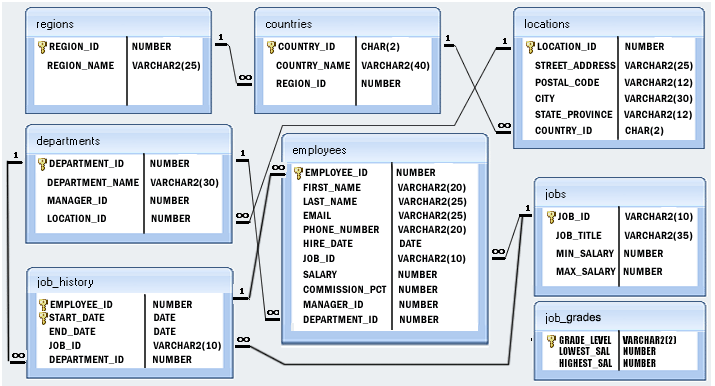﻿ Pandas HR database: Select distinct department id from employees file - w3resource# Pandas HR database: Select distinct department id from employees file

## Pandas HR database Queries: Exercise-4 with Solution

Write a Pandas program to select distinct department id from employees file.

Sample Solution :

Python Code :

``````import pandas as pd
print("Distinct department_id:")
print(employees.department_id.unique())
``````

Sample Output:

``` Distinct department_id:
[ 90.  60. 100.  30.  50.  80.  nan  10.  20.  40.  70. 110.]
```

Equivalent SQL Syntax:

```SELECT DISTINCT department_id FROM EMPLOYEES;
```

Click to view the table contain:

Employees Table

Departments Table

Countries Table

Job_History Table

Jobs Table

Locations Table

Regions Table

Python Code Editor:

Structure of HR database :Have another way to solve this solution? Contribute your code (and comments) through Disqus.

What is the difficulty level of this exercise?

﻿

## Python: Tips of the Day

Checks if the given number falls within the given range.

Example:

```def tips_range(n, start, end = 0):
return start <= n <= end if end >= start else end <= n <= start
print(tips_range(2, 4, 6))
print(tips_range(4, 8))
print(tips_range(1, 3, 5))
print(tips_range(1, 3))
```

Output:

```False
True
False
True
```